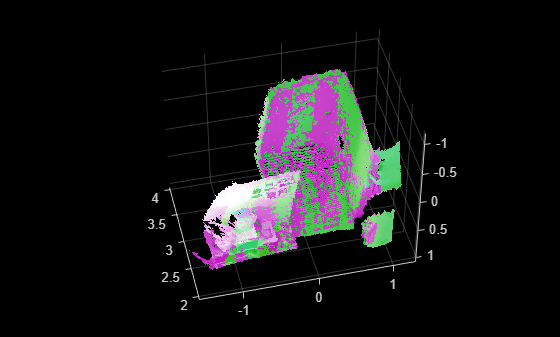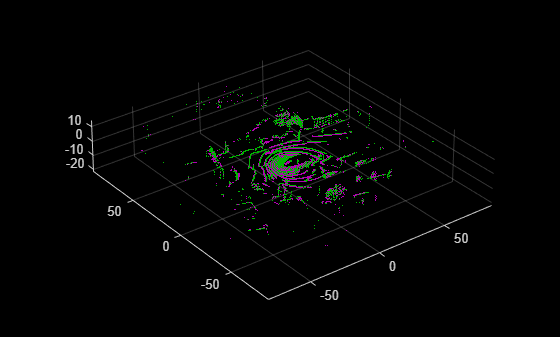# pcregisterloam

Register two point clouds using LOAM algorithm

Since R2022a

## Syntax

``tform = pcregisterloam(movingPtCloud,fixedPtCloud,gridStep)``
``tform = pcregisterloam(movingPoints,fixedPoints)``
``[tform,rmse] = pcregisterloam(___)``
``[___] = pcregisterloam(___,Name=Value)``

## Description

example

````tform = pcregisterloam(movingPtCloud,fixedPtCloud,gridStep)` registers the moving point cloud `movingPoints` with the fixed point cloud `fixedPoints` using the lidar odometry and mapping (LOAM) algorithm. The function returns the rigid transformation `tform`, between the moving and fixed point clouds. `gridStep` specifies the size of a 3-D box used to downsample the LOAM points detected in the input point clouds.```
````tform = pcregisterloam(movingPoints,fixedPoints)` registers the moving LOAM points `movingPoints` with the fixed LOAM points `fixedPoints` and returns the rigid transformation between them `tform`. Using this function to register LOAM points is faster and more accurate than using it to register point clouds.You can obtain the LOAM points of the moving and fixed point clouds by using the `detectLOAMFeatures` function, which detects LOAM feature points from organized point clouds. ```
````[tform,rmse] = pcregisterloam(___)` returns the root mean squared error `rmse` of the Euclidean distance between the aligned points.```
````[___] = pcregisterloam(___,Name=Value)` specifies options using one or more name-value arguments in addition to any combination of arguments from previous syntaxes. For example, `MatchingMethod`=`'one-to-one'` sets the matching method algorithm to `'one-to-one'`.```

## Examples

collapse all

Load and visualize point cloud data.

```ld = load('livingRoom.mat'); movingPtCloud = ld.livingRoomData{1}; fixedPtCloud = ld.livingRoomData{2}; figure pcshowpair(movingPtCloud,fixedPtCloud,'VerticalAxis','Y','VerticalAxisDir','Down')```Register the point clouds.

```gridStep = 0.5; tform = pcregisterloam(movingPtCloud,fixedPtCloud,gridStep);```

Align and visualize the point clouds.

```alignedPtCloud = pctransform(movingPtCloud, tform); figure pcshowpair(alignedPtCloud,fixedPtCloud,'VerticalAxis','Y','VerticalAxisDir','Down')```Read point cloud data from a Velodyne PCAP file into the workspace.

`veloReader = velodyneFileReader("lidarData_ConstructionRoad.pcap","HDL32E");`

Read the first point cloud from the data. Use this point cloud as the fixed point cloud.

`fixedPtCloud = readFrame(veloReader,1);`

Detect LOAM feature points in the fixed point cloud.

`fixedPoints = detectLOAMFeatures(fixedPtCloud);`

Downsample the less planar surface points from the fixed point cloud, to improve registration speed.

```gridStep = 1; fixedPoints = downsampleLessPlanar(fixedPoints,gridStep);```

Read and detect LOAM feature points from the fifth point cloud in the data. Use this point cloud as the moving point cloud.

```movingPtCloud = readFrame(veloReader,5); movingPoints = detectLOAMFeatures(movingPtCloud);```

Downsample the less planar surface points from the moving point cloud.

`movingPoints = downsampleLessPlanar(movingPoints,gridStep);`

Register the moving point cloud to the fixed point cloud.

`tform = pcregisterloam(movingPoints,fixedPoints);`

Transform the moving point cloud to align it to the fixed point cloud.

`alignedPtCloud = pctransform(movingPtCloud,tform);`

Visualize the aligned point clouds. Points from the fixed point cloud display as green, while points from the moving point cloud display as magenta.

```figure pcshowpair(alignedPtCloud,fixedPtCloud)```## Input Arguments

collapse all

Organized moving point cloud, specified as a `pointCloud` object. The point cloud object must contain an organized point cloud with a `Location` property of size M-by-N-by-3 matrix, where M is the number of laser scans and N is the number of points per scan. The first page represents the x-coordinates, the second page represents the y-coordinates, and the third page represents the z- coordinates for each point.

Organized fixed point cloud, specified as a `pointCloud` object. The point cloud object must contain an organized point cloud with a `Location` property of size M-by-N-by-3 matrix, where M is the number of laser scans and N is the number of points per scan. The first page represents the x-coordinates, the second page represents the y-coordinates, and the third page represents the z- coordinates for each point.

Moving LOAM points, specified as a `LOAMPoints` object. You can obtain the LOAM points from the moving point cloud by using the `detectLOAMFeatures` function, which detects LOAM feature points from organized point clouds.

Fixed LOAM points, specified as a `LOAMPoints` object. You can obtain the LOAM points from the fixed point cloud by using the `detectLOAMFeatures` function, which detects LOAM feature points from organized point clouds.

Size of the 3-D box for downsampling the detected LOAM points in the input point cloud, specified as a positive scalar.

### Name-Value Arguments

Specify optional pairs of arguments as `Name1=Value1,...,NameN=ValueN`, where `Name` is the argument name and `Value` is the corresponding value. Name-value arguments must appear after other arguments, but the order of the pairs does not matter.

Example: `pcregisterloam(movingPoints,fixedPoints,MatchingMethod='one-to-one')` sets the registration matching method to `'one-to-one'`.

Initial rigid transformation, specified as a `rigidtform3d` object. Set the initial transformation to a coarse estimate. If you do not provide a coarse or initial estimate, the function uses a `rigidtform3d` object that contains a translation that moves the center of the moving points to the center of the fixed points.

Matching method, specified as `'one-to-one'` or `'one-to-many'`.

• `'one-to-one'` — The algorithm selects the nearest neighbor as a match.

• `'one-to-many'` — The algorithm selects multiple nearest neighbors within the specified search radius as matches. Using the `'one-to-many'` method can increase registration accuracy, but at the cost of processing speed.

Search radius for point matching, specified as a positive integer. When matching, the function finds the closest edge and surface points within the specified radius. For best results, set this value based on the certainty of the `InitialTransform`. Increase the value of the `SearchRadius` when there is greater uncertainty in the initial transformation, but this can also decrease the registration speed.

Maximum iterations before LOAM registration stops, specified as a positive integer.

Tolerance between consecutive LOAM iterations, specified as a two-element vector with nonnegative values. The vector, [Tdiff Rdiff].

• Tdiff — Tolerance for the estimated absolute difference in translation and rotation estimated in consecutive LOAM iterations. Measures the Euclidean distance between two translation vectors.

• Rdiff — Tolerance for the estimated absolute difference of the angular rotation between consecutive iterations, measured in degrees.

The algorithm stops when the difference between the estimates of the rigid transformations in the most recent consecutive iterations falls below the specified tolerance value.

Display progress information, specified as a numeric or logical `0` (`false`) or `1` (`true`). To display progress information, set `Verbose` to `true`.

## Output Arguments

collapse all

Rigid 3-D transformation, returned as a `rigidtform3d` object. `tform` describes the rigid 3-D transformation that registers the moving points to the fixed points.

Root mean squared error, returned as a positive scalar that represents the Euclidean distance between aligned points. A lower `rmse` value indicates a more accurate registration.

 Zhang, Ji, and Sanjiv Singh. “LOAM: Lidar Odometry and Mapping in Real-Time.” In Robotics: Science and Systems X. Robotics: Science and Systems Foundation, 2014. https://doi.org/10.15607/RSS.2014.X.007.